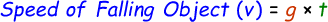# Speed of Falling Object Calculator

With the help of our online speed of falling object calculator you will be able to find the speed at which the body is falling onto the ground. This can be with the help of a free fall formula:where,
v = Velocity [m/s]
g = Gravitational constant [m/s2]
t = Time taken [sec]

Example: A ball is dropped onto the floor from a building terrace. It took 15 seconds for the ball to land onto the floor. Calculate the speed at which the ball traveled before landing.
Solution:
Given: t = 15 seconds

We know the formula to calculate speed of falling object:

v = g x t

= 9.8 x 15

= 147

Hence, the speed of the ball before landing onto the ground is 147 m/s.

In the below gravity speed calculator, enter the input values and click calculate button to find the answer.

 Time Taken (t): [sec] Gravity (g): [m/s^2] Speed of Falling Object: [m/s]

Latest Calculator Release

Average Acceleration Calculator

Average acceleration is the object's change in speed for a specific given time period. ...

Free Fall Calculator

When an object falls into the ground due to planet's own gravitational force is known a...

Torque Calculator

Torque is nothing but a rotational force. In other words, the amount of force applied t...

Average Force Calculator

Average force can be explained as the amount of force exerted by the body moving at giv...

Angular Displacement Calculator

Angular displacement is the angle at which an object moves on a circular path. It is de...